International
Tables for
Crystallography
Volume H
Powder diffraction
Edited by C. J. Gilmore, J. A. Kaduk and H. Schenk

International Tables for Crystallography (2018). Vol. H, ch. 3.4, pp. 272-275

## Section 3.4.3. Indexing methods

A. Altomare,a* C. Cuocci,a A. Moliternia and R. Rizzia

aInstitute of Crystallography – CNR, Via Amendola 122/o, Bari, I-70126, Italy
Correspondence e-mail:  angela.altomare@ic.cnr.it

### 3.4.3. Indexing methods

| top | pdf |

Indexing methods aim to reconstruct the three-dimensional direct lattice from the one-dimensional distribution of dhkl values. Systematic or accidental peak overlap, inaccuracy of peak positions, zero shift in the 2θhkl Bragg angles and/or the presence of impurity peaks make the reconstruction difficult. Data accuracy is fundamental for increasing the probability of success; as emphasized by de Wolff: The indexing problem' is essentially a puzzle: it cannot be stated in rigorous terms (…). It would be quite an easy puzzle if errors of measurements did not exist' (de Wolff, 1957).

Different approaches have been proposed for solving the indexing puzzle since the pioneering work of Runge (1917). As suggested by Shirley (2003), indexing procedures work in parameter space, or in index space, or in both spaces. As a general consideration, the parameter space allows the inclusion of the cell information and constraints, while the index space is more suitable in cases where there are accidental or systematic absences (Shirley, 1980). In this section an outline of the strategies and search methods adopted by the main traditional and non-traditional indexing approaches is given. For more details see the papers by Shirley (2003) and Bergmann et al. (2004).

Among the main indexing procedures, zone indexing (Section 3.4.3.1.1), SIW heuristic (Section 3.4.3.1.2), successive dichotomy (Section 3.4.3.1.5), the topographs method (Section 3.4.3.2.1) and global-optimization methods (Section 3.4.3.2.2) operate in the parameter space; index heuristics (Section 3.4.3.1.3) and index permutation (Section 3.4.3.1.4) work in the index space; and scan/covariance (Bergmann, 2007) operates both in index and parameter space. Each method can be classified as exhaustive or not. An exhaustive method systematically and rigorously searches in the solution space; a non-exhaustive method exploits coincidences and relations between the observed lines with the aim of finding the solution quickly. The classification is not rigorous: approaches that try to combine rigour and speed can be defined as semi-exhaustive (Table 3.4.3).

 Table 3.4.3| top | pdf | Classification of indexing methods
Method (strategy/search)SpaceExhaustive
Zone indexing Parameter No
SIW heuristic Parameter Semi
Scan/covariance Parameter (for cubic to monoclinic); index (for triclinic) To monoclinic
Index heuristics Index Semi
Index permutation Index Yes
Successive dichotomy Parameter Yes
Grid search Parameter Yes
Genetic algorithms Parameter No
Monte Carlo Parameter No

Indexing procedures can also be classified as traditional and non-traditional. Each indexing method generates a list of possible cells. Their reliability is assessed by FOMs with the aim of selecting the correct one (see Section 3.4.2.1).

#### 3.4.3.1. Traditional indexing methods

| top | pdf |

The traditional indexing approaches adopted over the last century are based on the following strategies and search methods: (1) zone indexing, (2) SIW heuristic, (3) index heuristics, (4) index permutation and (5) successive dichotomy. All of them exploit information about a limited number of observed peak positions.

#### 3.4.3.1.1. Zone-indexing strategy

| top | pdf |

The zone-indexing strategy was originally developed by Runge (1917), successively proposed by Ito (1949, 1950), generalized by de Wolff (1957, 1958) and enhanced by Visser (1969). This approach is based on the search for zones, i.e., crystallographic planes, in the reciprocal lattice, defined by the origin O and two lattice points. If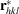and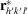are two vectors in reciprocal space, i.e. the positional vectors of the lattice points A and A′, they describe a zone containing any lattice point B whose positional vector is of type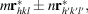where m and n are positive integers. If ω is the angle betweenand, the squared distance of B from O (i.e.,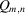) can be expressed by (de Wolff, 1958; Visser, 1969)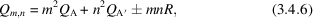where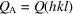and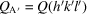are the squared distances of A and A′ from O, respectively, and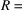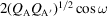. R can be derived as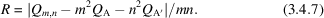The method is applied as follows:and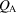are chosen among the first experimental Qi values; the {Qi}, up to a reasonable resolution, are introduced in (3.4.7)in place of; and a few positive integer values are assigned to m and n. Equation (3.4.7)provides a large number of R values; equal R values (within error limits) define a zone, for which the ω angle can easily be calculated. The search for zones is performed using different () pairs. The R values that are obtained many times identify the most important crystallographic zones. The zones are sorted according to a quality figure, enabling selection of the best ones. In order to find the lattice, all possible combinations of the best zones are tried. For every pair of zones the intersection line is found, then the angle between them is determined and the lattice is obtained.

The method has the advantage of being very efficient for indexing low-symmetry patterns. The main disadvantage is its sensitivity to errors in the peak positions, particularly in the low 2θ region.

#### 3.4.3.1.2. Shirley–Ishida–Watanabe (SIW) heuristic strategy

| top | pdf |

This needs only one single well-established zone, then it arbitrarily chooses the 001 line from the first-level lines. The indexing problem is thus lowered to two dimensions and an exhaustive search is carried out.

#### 3.4.3.1.3. Index-heuristics strategy

| top | pdf |

The index-heuristics strategy searches for the correct cell via a trial-and-error approach, assigning tentative Miller indices to a few experimental peak positions (basis lines), usually belonging to the low 2θ region of the experimental pattern. It was first proposed by Werner (1964), then successively refined (Werner et al., 1985) and made more robust and effective (Altomare et al., 2000, 2008, 2009). This approach, which works in the index space, was defined by Shirley as semi-exhaustive (Shirley, 1980). The search starts from the highest-symmetry crystal system (cubic) and, if no plausible solution is found, it is extended to lower symmetry down to triclinic. The number of selected basis lines increases as the crystal symmetry lowers. A dominant zone occurs when one cell axis is significantly shorter than the other two; in this case most of the first observed lines (in terms of increasing 2θhkl values) can be indexed with a common zero Miller index. Special short-axis tests, aimed at finding two-dimensional lattices, have been proposed for monoclinic symmetry in order to detect the presence of dominant zones (Werner et al., 1985). The index-heuristics method is based on the main indexing equation [see equation (3.4.2)] that can be rewritten (Werner et al., 1985) as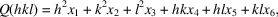where {xi} = X is the vector of unknown parameters, which are derived by solving a system of linear equations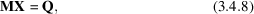where M is a matrix of Miller indices and Q is the vector of the selected Q(hkl) values corresponding to the basis lines. The dimensions of M, X and Q change according to the assumed symmetry. From the inverse matrix M−1 the corresponding X is obtained via X = M−1Q. In the case of monoclinic and higher symmetry, {xi} are calculated by Cramer's rule. Different X vectors are derived by using a different selection of basis lines. The possible solutions are checked by using the full list of peak positions (up to the first 25 experimental lines). The method is sensitive to errors on peak positions and to the presence of impurities (the presence of only one impurity peak is not critical). The correctness of the {xi} strongly depends on the accuracy of the observed Q values, especially for low-2θ region lines, which are the most dominant ones for this indexing procedure. The possibility of testing different combinations of basis-line sets enables the correct cell to be found by bypassing the cases for which errors in the basis lines occur.

The method has been recently enhanced (Altomare et al., 2000, 2009) by introducing new procedures that are able to increase the probability of successful indexing (see Section 3.4.4.2.1); among them are: (1) a correction for systematic errors in the experimental 2θ values (positive and negative trial 2θ zero shifts are taken into account); this correction should, in principle, describe a real diffractometer error; in practice, it also approximates the specimen displacement error well (perhaps coupled with transparency for organic samples); (2) a more intensive search in solution space for orthorhombic and monoclinic systems; (3) an improvement of the triclinic search; (4) a new figure of merit, WRIP20, which is more powerful than M20 in identifying the correct solution among a set of possible ones (see Section 3.4.2.1); (5) a check for geometrical ambiguities; (6) an automatic refinement of the possible cells; and (7) a statistical study of the parity of the Miller indices, performed at the end of the cell refinement, aimed at detecting doubled axes or additional lattice points (for A-, B-, C-, I-, R- or F-centred cells) (such information is used in the successive steps).

#### 3.4.3.1.4. Index-permutation strategy

| top | pdf |

This strategy was proposed by Taupin (1973), and is based on a systematic permutation of indices associated to observed lines for obtaining candidate cells. Because this trial-and-error strategy is similar to the index-heuristics approach, we do not describe it here.

#### 3.4.3.1.5. Successive-dichotomy search method

| top | pdf |

The successive-dichotomy method, first developed by Louër & Louër (1972), is based on an exhaustive strategy working in direct space (except for triclinic systems, where it operates in reciprocal space) by varying the lengths of the cell axes and the interaxial angles within finite intervals. The search for the correct cell is performed in an n-dimensional domain D (where n is the number of cell parameters to be determined). If no solution belongs to D, the domain is discarded and the ranges for the allowed values of cell parameters are increased; on the contrary, if D contains a possible solution, it is explored further by dividing the domain into 2n subdomains via a successive-dichotomy procedure. Each subdomain is analyzed and discarded if it does not contain a solution. The method was originally applied to orthorhombic and higher-symmetry systems (Louër & Louër, 1972), but it has been successively extended to monoclinic (Louër & Vargas, 1982) and to triclinic systems (Boultif & Louër, 1991). The search can be performed starting from cubic then moving down to lower symmetries (except for triclinic) by partitioning the space into shells of volume ΔV = 400 A3. For triclinic symmetry ΔV is related to the volume Vest suggested by the method proposed by Smith (1977), which is able to estimate the unit-cell volume from only one line in the pattern: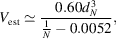where dN is the value for the Nth observed line; in the case N = 20 the triclinic cell volume is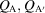.

Let us consider, as an example, the monoclinic case; in terms of direct cell parameters, Q(hkl) is given by (Boultif & Louër, 1991)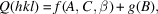where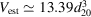,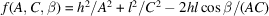,,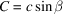and B = b. The search using the successive-dichotomy method is performed in a four-dimensional space that is covered by increasing the integer values i, l, m and n in the intervals [A, A+] = [A = A0 + ip, A+ = A + p], [B, B+] = [B = B0 + lp, B+ = B + p], [C, C+] = [C = C0 + mp, C+ = C + p] and [β, β+] = [β = 90 + nθ, β+ = β + θ], where the step values of p and θ are 0.4 Å and 5°, respectively, and A0, B0 and C0 are the lowest values of A, B and C (based on the positions of the lowest-angle peaks), respectively. Each quartet of intervals defines a domain D and, by taking into account the current limits for the parameters A, B, C and β, a calculated pattern is generated, not in terms of discrete Q(hkl) values but of allowed intervals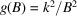. D is retained only if the observed Qi values belong to the range [Q(hkl) − ΔQi, Q+(hkl) + ΔQi], where ΔQi is the absolute error of the observed lines (i.e., impurity lines are not tolerated). If D has been accepted, it is divided into 24 subdomains by halving the original intervals [A, A+], [B, B+], [C, C+] and [β, β+] and new limitsare calculated; if a possible solution is found, the dichotomy method is applied iteratively. In case of triclinic symmetry the expression for Q(hkl) in terms of direct cell parameters is too complicated to be treated via the successive-dichotomy method; therefore the basic indexing equation (3.4.2)is used. In this case, the [Q(hkl), Q+(hkl)] intervals are set in reciprocal space according to the Aij parameters of (3.4.2). To reduce computing time the following restrictions are put on the (hkl) Miller indices associated with the observed lines: (1) maximum h, k, l values equal to 2 in case of the first five lines; (2) h + k + l < 3 for the first two lines.

The outcome of the successive-dichotomy method is not strongly influenced by the presence of a dominant zone. New approaches have been devoted to overcome the limitations of the method with a strict dependence on data accuracy and on impurities (Boultif & Louër, 2004; Louër & Boultif, 2006, 2007), see Section 3.4.4.2).

#### 3.4.3.2. Non-traditional indexing methods

| top | pdf |

New indexing procedures that provide alternatives to the traditional approaches outlined in Section 3.4.3.1have recently been proposed.

#### 3.4.3.2.1. The topographs method

| top | pdf |

This method (Oishi et al., 2009) is based on the Ito equation (de Wolff, 1957):where Q(h) is the length of the reciprocal vectorcorresponding to the Miller index vector h = (hkl). It uses Conway's topograph (Conway & Fung, 1997), a connected tree obtained by associating a graph to each equation of type (3.4.9)and consisting of infinite directed edges. According to Ito's method, if quadrupoles (Q1, Q2, Q3, Q4) detected among the observed Qi values satisfy the condition 2(Q1 + Q2) = Q3 + Q4, two Miller-index vectors h1 and h2 are expected to exist such that Q1 = Q(h1), Q2 = Q(h2), Q3 = Q(h1h2) and Q4 = Q(h1 + h2). If an additional value Q5 satisfying the condition 2(Q1 + Q4) = Q2 + Q5 is found, the graph of the quadrupole (Q1, Q2, Q3, Q4) grows via the addition of the Q5 contribution; this procedure is iterated. If topographs share a Q value that corresponds to the same reciprocal-lattice vector, then a three-dimensional lattice is derived containing the two-dimensional lattices associated with the original topographs. Three-dimensional lattices are also obtained by combining topographs. The probability that topographs correspond to the correct cell increases with the number of edges of the graph structure. The method is claimed by the authors to be insensitive to the presence of impurity peaks.

#### 3.4.3.2.2. Global-optimization methods

| top | pdf |

Global-optimization methods, widely adopted for solving crystal structures from powder data, have also been successfully applied to indexing. Among them, we provide brief descriptions of genetic algorithms, and Monte Carlo and grid-search methods.

#### 3.4.3.2.2.1. Genetic-algorithm search method

| top | pdf |

The use of genetic algorithms (GAs) for solving the indexing problem was proposed by Tam & Compton (1995) and Paszkowicz (1996). Since then, Kariuki and co-workers (Kariuki et al., 1999) have combined GAs with a whole-profile-fitting procedure for indexing powder diffraction patterns. This approach exploits the information of the full powder diffraction pattern. It is inspired by the Darwinian evolutionary principle based on mating, mutation and natural selection of the member of a population that survives and evolves to improve future generations. The initial population consists of a set of trial cell parameters, chosen randomly within a given volume range; a full pattern-decomposition process is performed using the Le Bail algorithm (Chapter 3.5) and the agreement between the calculated and observed profiles is derived and used for assessing the goodness of an individual member (i.e., a set of unit-cell parameters). The most plausible cell is therefore found by exploring a six-dimensional hypersurfaceand searching for the global minimum of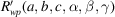(see Section 3.4.4.3.2). In contrast to the main traditional methods, whose outcomes depend on the reliability of a set of peak positions, this procedure has the advantage of being insensitive to the presence of small impurity peaks that have a negligible influence on the agreement factor between the experimental and calculated profiles: the global minimum ofis reached if the majority phase is correctly indexed. The main disadvantage of the method is the computing time required, in particular in the case of low symmetry.

#### 3.4.3.2.2.2. Monte Carlo search method

| top | pdf |

The Monte Carlo approach has also been applied to indexing powder diffraction patterns (Le Bail, 2004; Bergmann et al., 2004; Le Bail, 2008). It exploits all the information contained in the full pattern, randomly generates and selects trial cell parameters, and calculates peak positions to which it assigns the corresponding Miller indices. An idealized powder pattern consisting of peak positions d and extracted intensities I is considered to test the trial cell. The cell reliability is assessed by suitable figures of merit (e.g. Rp and McM20, see Section 3.4.2.1). The main drawback of this approach is the significant computing time required, in particular for triclinic systems.

#### 3.4.3.2.2.3. Grid-search method

| top | pdf |

This performs an iterated `step-and-repeat search' in the parameter space. It has the advantage of being flexible, exhaustive and not particularly sensitive to impurities or errors, and the disadvantage of being slow (Shirley, 2003).

### References

Altomare, A., Campi, G., Cuocci, C., Eriksson, L., Giacovazzo, C., Moliterni, A., Rizzi, R. & Werner, P.-E. (2009). Advances in powder diffraction pattern indexing: N-TREOR09. J. Appl. Cryst. 42, 768–775.Google Scholar
Altomare, A., Giacovazzo, C., Guagliardi, A., Moliterni, A. G. G., Rizzi, R. & Werner, P.-E. (2000). New techniques for indexing: N-TREOR in EXPO. J. Appl. Cryst. 33, 1180–1186.Google Scholar
Altomare, A., Giacovazzo, C. & Moliterni, A. (2008). Indexing and space group determination. In Powder Diffraction Theory and Practice, edited by R. E. Dinnebier & S. J. L. Billinge, pp. 206–226. Cambridge: RSC Publishing.Google Scholar
Bergmann, J. (2007). EFLECH/INDEX – another try of whole pattern indexing. Z. Kristallogr. Suppl. 26, 197–202.Google Scholar
Bergmann, J., Le Bail, A., Shirley, R. & Zlokazov, V. (2004). Renewed interest in powder diffraction data indexing. Z. Kristallogr. 219, 783–790.Google Scholar
Boultif, A. & Louër, D. (1991). Indexing of powder diffraction patterns for low-symmetry lattices by the successive dichotomy method. J. Appl. Cryst. 24, 987–993.Google Scholar
Boultif, A. & Louër, D. (2004). Powder pattern indexing with the dichotomy method. J. Appl. Cryst. 37, 724–731.Google Scholar
Buerger, M. J. (1957). Reduced cells. Z. Kristallogr. 109, 42–60.Google Scholar
Conway, J. H. & Fung, F. Y. C. (1997). The Sensual (Quadratic) Form. Washington, DC: The Mathematical Association of America.Google Scholar
Ito, T. (1949). A general powder X-ray photography. Nature, 164, 755–756.Google Scholar
Ito, T. (1950). X-ray Studies on Polymorphism. Tokyo: Maruzen Company.Google Scholar
Kariuki, B. M., Belmonte, S. A., McMahon, M. I., Johnston, R. L., Harris, K. D. M. & Nelmes, R. J. (1999). A new approach for indexing powder diffraction data based on whole-profile fitting and global optimization using a genetic algorithm. J. Synchrotron Rad. 6, 87–92.Google Scholar
Le Bail, A. (2004). Monte Carlo indexing with McMaille. Powder Diffr. 19, 249–254.Google Scholar
Le Bail, A. (2008). Structure solution. In Principles and Applications of Powder Diffraction, edited by A. Clearfield, J. H. Reibenspies & N. Bhuvanesh, pp. 261–309. Oxford: Wiley-Blackwell.Google Scholar
Louër, D. & Boultif, A. (2006). Indexing with the successive dichotomy method, DICVOL04. Z. Kristallogr. Suppl. 23, 225–230.Google Scholar
Louër, D. & Louër, M. (1972). Méthode d'essais et erreurs pour l'indexation automatique des diagrammes de poudre. J. Appl. Cryst. 5, 271–275.Google Scholar
Louër, D. & Vargas, R. (1982). Indexation automatique des diagrammes de poudre par dichotomies successives. J. Appl. Cryst. 15, 542–545.Google Scholar
Oishi, R., Yonemura, M., Hoshikawa, A., Ishigaki, T., Mori, K., Torii, S., Morishima, T. & Kamiyama, T. (2009). New approach to the indexing of powder diffraction patterns using topographs. Z. Kristallogr. Suppl. 30, 15–20.Google Scholar
Paszkowicz, W. (1996). Application of the smooth genetic algorithm for indexing powder patterns – tests for the orthorhombic system. Mater. Sci. Forum, 228–231, 19–24.Google Scholar
Runge, C. (1917). Die Bestimmung eines Kristallsystems durch Rontgenstrahlen. Phys. Z. 18, 509–515.Google Scholar
Shirley, R. (1980). Data accuracy for powder indexing. In Accuracy in Powder Diffraction, edited by S. Block & C. R. Hubbard, NBS Spec. Publ. 567, 361–382.Google Scholar
Shirley, R. (2003). Overview of powder-indexing program algorithms (history and strengths and weaknesses). IUCr Comput. Comm. Newsl. 2, 48–54. http://www.iucr.org/resources/commissions/crystallographic-computing/newsletters/2 .Google Scholar
Smith, G. S. (1977). Estimating the unit-cell volume from one line in a powder diffraction pattern: the triclinic case. J. Appl. Cryst. 10, 252–255.Google Scholar
Tam, K. Y. & Compton, R. G. (1995). GAMATCH – a genetic algorithm-based program for indexing crystal faces. J. Appl. Cryst. 28, 640–645.Google Scholar
Taupin, D. (1973). A powder-diagram automatic-indexing routine. J. Appl. Cryst. 6, 380–385.Google Scholar
Visser, J. W. (1969). A fully automatic program for finding the unit cell from powder data. J. Appl. Cryst. 2, 89–95.Google Scholar
Werner, P.-E. (1964). Trial-and-error computer methods for the indexing of unknown powder patterns. Z. Kristallogr. 120, 375–387.Google Scholar
Werner, P.-E., Eriksson, L. & Westdahl, M. (1985). TREOR, a semi-exhaustive trial-and-error powder indexing program for all symmetries. J. Appl. Cryst. 18, 367–370.Google Scholar
Wolff, P. M. de (1957). On the determination of unit-cell dimensions from powder diffraction patterns. Acta Cryst. 10, 590–595.Google Scholar
Wolff, P. M. de (1958). Detection of simultaneous zone relations among powder diffraction lines. Acta Cryst. 11, 664–665.Google Scholar